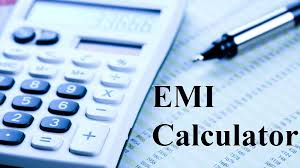# Here’s How Calculate Personal Loan Emi In 3 Easy Steps?When it comes to borrowing funds, people like to avail a personal loan to manage their unexpected financial needs. A personal loan is one of the most viable options for the borrowers due to its easy availability, paperless documentation, flexible tenure, and affordable rate of interests. Further, such loans are unsecured in nature, which means that the applicant does not have to provide any asset to the lender as security.

Not all lenders provide the same offers regarding a personal loan. If you are considering to take a loan, it is necessary to compare the options provided by various lending institutions. You must choose the offer that provides low-interest rates, flexible tenure, and reasonable Equated Monthly Installments (EMIs) that do not deplete your current savings. Hence, it is better to use the personal loan EMI calculator to know the EMI amount and choose the one which suits you.

## Calculation of personal loan EMI

EMI, a part of the loan you have availed, is the fixed amount that a borrower is required to pay to the lender on a specified date each month. It mainly consists of two components – the interest and the principal. The EMI assessment is influenced by three crucial variables:

• Interest rate –  It is calculated based on the outstanding loan amount.
• Loan amount – This is the value of the loan that you want to secure. Higher the amount, lower the EMI payments.
• Tenure of the loan – The period for which you avail the loan. Longer loan tenure means smaller EMIs and vice versa.

### 1. Using the Personal loan EMI Calculator

Personal loan EMI Calculator is a financial tool that assists in computing the monthly loan instalments, i.e., EMIs of your loan. Every lender provides an EMI calculator on its official website. It is super flexible to use and gives reliable results based on relevant information, such as personal loan interest rates, loan amount, and tenure of the loan. With this online calculator, you can get an idea of how much loan you can afford to take and plan your borrowings and repayments as per your requirements.

Usually, PA EMI calculators have a slider on the range of each of the three variables – interest rate, the loan amount that suits your budget and rate of interest, and tenure of the repayment of the loan. Here, the rate of interest, which is provided by the NBFCs, is fixed, whereas, you can select any loan amount and tenure according to your financial needs.

Follow these three simple steps to compute EMI using a personal loan EMI calculator:

• Use the slider to enter the loan amount you wish to secure, the rate of interest imposed by the lender, and the loan period over which you decide to repay the loan.
• The calculator will immediately showcase the different EMIs for different loan term and amount.
• If you are not satisfied with the EMI, you can input varying loan tenure and value until you find the most affordable amount as per your needs. For example, if the EMI is high than expected, you can select a smaller loan amount and longer loan tenure. On the contrary, entering a larger loan amount and shorter tenure can be useful in case of low EMI value.

### 2. Using PMT formula

PMT formula is another effective way of assessing the EMIs. As compared to the online calculator, it involves long and tedious calculations and requires Microsoft Excel to run.

The formula is:

PMT (Rate, NPER, PV)

Where

• PMT is the EMI
• NPER means the total number of payments for the loan.
• PV is the present loan value.

You can use this formula with different combinations and then pick the one that is the most affordable as per your requirements.

### 3. Using mathematical formula

You can also apply a mathematical formula to know the EMI value for your loan. If you use this method, you will get the same result that you will get in the PMT formula.

The formula is as follows:

EMI = [P x R x (1+R) N]/[(1+R) N-1]

• P is the loan amount or principal
• R is the interest rate per month
• N is the number of monthly instalments

## Final thoughts

EMI is a significant factor, and calculating them before obtaining a personal loan can help the borrower in strategizing a repayment schedule for smooth reimbursement of the loan.